Courses

# Bending and Shear Stresses Civil Engineering (CE) Notes | EduRev

## Civil Engineering (CE) : Bending and Shear Stresses Civil Engineering (CE) Notes | EduRev

The document Bending and Shear Stresses Civil Engineering (CE) Notes | EduRev is a part of the Civil Engineering (CE) Course Topic wise GATE Past Year Papers for Civil Engineering.
All you need of Civil Engineering (CE) at this link: Civil Engineering (CE)

Q.1  For a channel section subjected to a downward vertical shear force at its centroid, which one of the following represents the correct distribution of shear stress in flange and web?    [2019 : 1 Mark, Set-ll]
(a)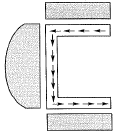(b)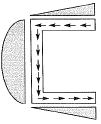(c)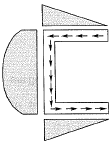(d)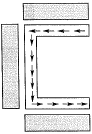Ans.
(C)
Solution:
Shear flow distribution for channel.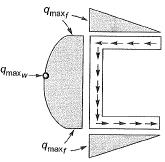Q.2 Cross section of a built-up wooden beam as shown in figure (not drawn to scale) is subjected to a vertical shear force of 8 kN. The beam is symmetrical about the neutral axis (NA), shown, and the moment of inertia about N.A. is 1.5 x 109 mm4. Considering that the nails at the location P are spaced longitudinally (along the length of the beam) at 60 mm, each of the nails at P will be subjected to the shear force of    [2019 : 2 Marks, Set-I]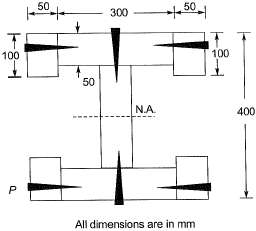(a) 240 N
(b) 480 N
(c) 60 N
(d) 120 N
Ans.
(A)
Solution: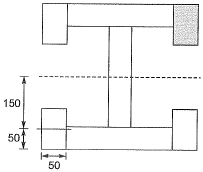Shear Flow,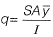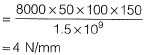Distance between two nails l = 60 mm
∴ S.F. resisted by each nail = q x l = 240 N

Q.3 For a given loading on a rectangular plain concrete beam with an overall depth of 500 mm, the compressive strain and tensile strain developed at the extreme fibers are of the same magnitude of 2.5 x 10-4. The curvature in the beam cross-section (in m-1, round off to 3 decimal places), is _______.    [2019 : 1 Mark, Set-I]
Solution:

Given: D= 500 mm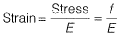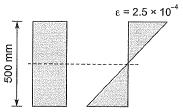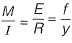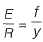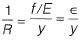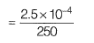= 1*10-6

Q.4 An 8 m long simply-supported elastic beam of rectangular cross-section (100 mm x 200 mm) is subjected to a uniformly distributed load of 10kN/m over its entire span. The maximum principal stress (in MPa, up to two decimal places) at a point located at the extreme compression edge of a cross-section and at 2 m from the support is ______ .    [2018 : 2 Marks, Set-II]
Solution: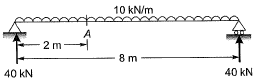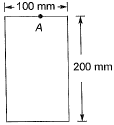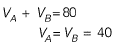[Due to symmetry]
MA = (-10 x 2 x 1) + 40 x 2
= 60 kNm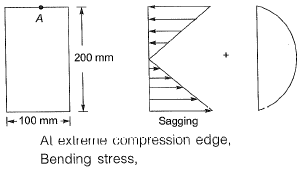σ = My/I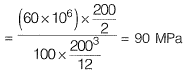Direct shear stress = 0
Principal stress,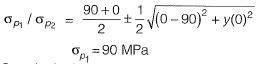So principal stress
= 90 N/mm2 = 90 MPa

Q.5 A cantilever beam of length 2 m with a square section of side length 0.1 m is loaded vertically at the free end. The vertical displacement at the free end is 5 mm. The beam is made of steel with Young’s modulus of 2.0 x 1011 N/m2. The maximum bending stress at the fixed end of the cantilever is    [2018 : 2 Marks, Set-I]
(a) 20.0 MPa
(b) 37.5 MPa
(c) 60.0 MPa
(d) 75.0 MPa
Ans.
(B)
Solution: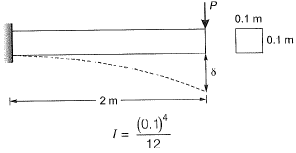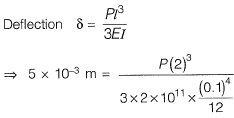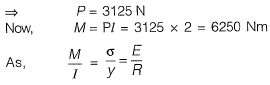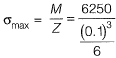= 37.5 x 106 N/m2 = 37.5 MPa

Q.6 A 450 mm long plain concrete prism is subjected to the concentrated vertical loads as shown in the figure. Cross section of the prism is given as 150 mm x 150 mm. Considering linear stress distribution across the cross-section, the modulus of rupture (expressed in MPa) is ___ .    [2016 : 2 Marks, Set-II]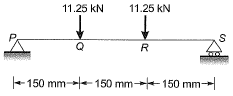Solution: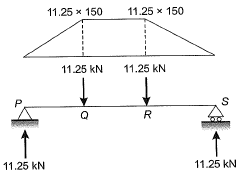BMQ = 11.25 x 150
= 1.6875 x 106 N-mm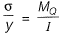where,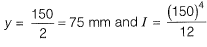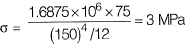Q.7 A simply supported reinforced concrete beam of length 10 m sags while undergoing shrinkage. Assuming a uniform curvature of 0.004 m-1 along the span, the maximum deflection (in m) of the beam at mid-span is ______ .    [2015 : 2 Marks, Set-II]
Solution: Method - I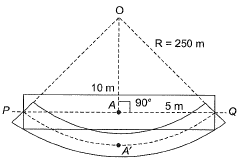Radius,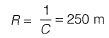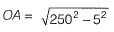= 249.95 m
Deflection = AA' = 250 - 249.95
= 0.05 m
Method-ll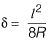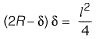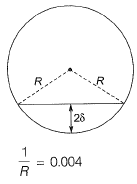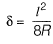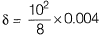δ 0.05 m
= 71.12N/mm2

Q.8 A symmetric l-section (with width of each flange = 50 mm, thickness of web = 10 mm) of steel is subjected to a shear force of 100 kN. Find the magnitude of the shear stress (in N/mm2) in the web at its junction with the top flange _____ .    [2013 : 1 Mark]
Solution: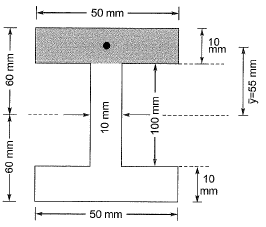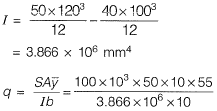Q.9 The “Plane section remain plane” assumption in bending theory implies    [2013 : 1 Mark]
(a) strain profile is linear
(b) stress profile is linear
(c) both profiles are linear
(d) shear deformation is neglected
Ans.
(A)
Solution: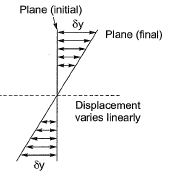Since, E ∝ δy
So, strain varies linearly.

Q.10 Consider a simply supported beam with a uniformly distributed load having a neutral axis (NA) as shown. For points P(on the neutral axis) and Q (at the bottom of the beam) the state of stress is best represented by which of the following pairs?    [2011 : 1 Mark]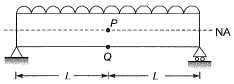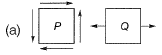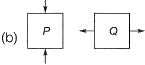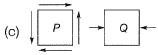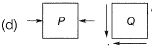Ans.
(B)
Solution: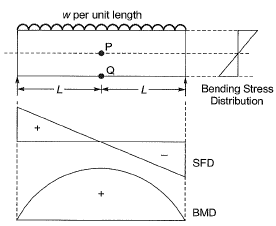Point P: Point Plies on NA, hence bending stress is zero at point P.
Point Palso lies at mid span, so shear force, V = 0
⇒ Shear stress, τ = 0
∴ State of stress of point Pwill be,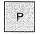Point Q: At point Q flexural stress is maximum and nature of which is tensile due to downward loading. Point Q lies at the extreme of beam, therefore, shear stress at point Q is zero.
∴ State of stress of point Q will be,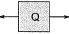Offer running on EduRev: Apply code STAYHOME200 to get INR 200 off on our premium plan EduRev Infinity!

69 docs

,

,

,

,

,

,

,

,

,

,

,

,

,

,

,

,

,

,

,

,

,

;